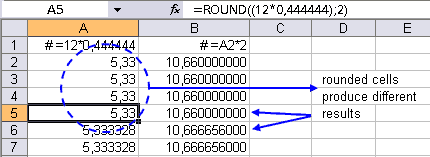## Round up formulas with =ROUND()I just got a call from a friend of mine. He needed to edit a spreadsheet and round a bunch of formulas. Since the rounding is important for the calculations, a simple cell-formatting wouldn't do. Excel's worksheet function =ROUND() can be used to round the numbers. However editing a lot of cells by hand (especially […]